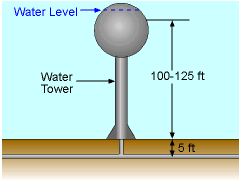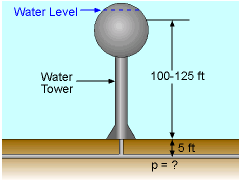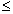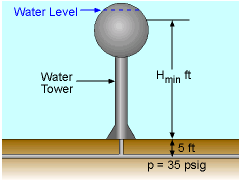Ch 2. Fluid Statics Multimedia Engineering Fluids PressureVariation PressureMeasurement HydrostaticForce(Plane) HydrostaticForce(Curved) Buoyancy
 Chapter 1. Basics 2. Fluid Statics 3. Kinematics 4. Laws (Integral) 5. Laws (Diff.) 6. Modeling/Similitude 7. Inviscid 8. Viscous 9. External Flow 10. Open-Channel Appendix Basic Math Units Basic Equations Water/Air Tables Sections Search eBooks Dynamics Fluids Math Mechanics Statics Thermodynamics Author(s): Chean Chin Ngo Kurt Gramoll ©Kurt GramollFLUID MECHANICS - EXAMPLE QuestionsWater Tower (a) Water towers in municipal distribution systems are often 100-125 ft in height. What pressure (psig) does this correspond to in the water pipes? (b) How far can the water level in the tank drop before the pressure in the water main drops below 35 psig? (Note, this is often the minimum design pressure.) SolutionProblem Diagram for Part (a) (a) Assume static conditions (reasonable since water in the tanks moves very slowly). Also, assume the water mains are buried 5 ft below grade. For hydrostatic conditions,      p = γH where γ = 62.4 lb/ft3 for water at 20 oC with H in ft, and p is in lb/ft2. Divide by 144 to get p in lb/in2 and the reference pressure is 0 atm (gage pressure), so      p = (62.4 lb/ft3)(H ft)(1 ft2/144 in2) For H = 100 + 5 = 105 ft: p = 45.5 psig For H = 125 + 5 = 130 ft: p = 56.3 psig Hence, the pressure range is       45.5 psigp56.3 psigProblem Diagram for Part (b) (b) Find H if p = 35 psig.      H = (35 psig)(144 in2/ft2) / 62.4 lb/ft3 = 80.8 ft Hence, the minimum water level in the tank is      Hmin = 80.8 - 5 = 75.8 ft Note: 2.31 ft static water = 1 psig pressure, which is a good conversion factor to remember.

Practice Homework and Test problems now available in the 'Eng Fluids' mobile app
Includes over 250 problems with complete detailed solutions.
Available now at the Google Play Store and Apple App Store.